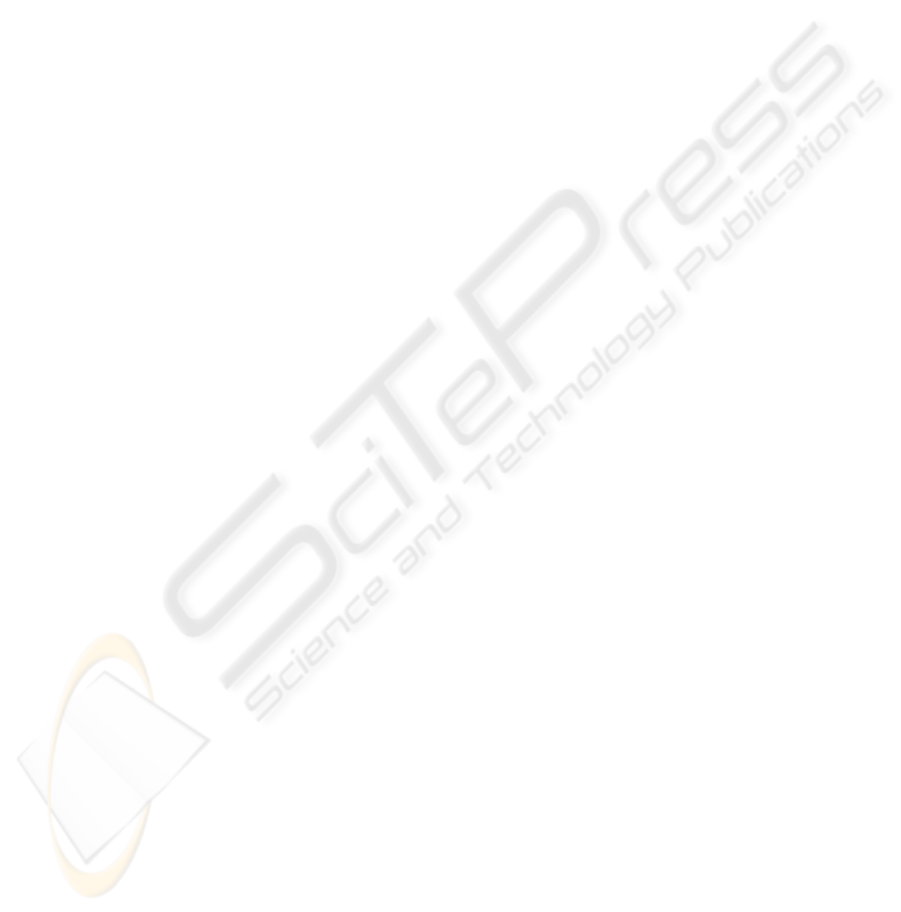FACIAL PARTS RECOGNITION USING LIFTING WAVELET
FILTERS LEARNED BY KURTOSIS-MINIMIZATION
Koichi Niijima
Department of Informatics, Kyushu University
6-1, Kasuga-koen, Kasuga, Fukuoka 816-8580, Japan
Keywords:
Facial parts recognition, lifting wavelet ﬁlter, learning, kurtosis, ill-posed problem, regularization.
Abstract:
We propose a method for recognizing facial parts using the lifting wavelet ﬁlters learned by kurtosis-
minimization. This method is based on the following three features of kurtosis: If a random variable has
a gaussian distribution, its kurtosis is zero. If the kurtosis is positive, the respective distribution is super-
gaussian. The value of kurtosis is bounded below. It is known that the histogram of wavelet coefﬁcients for a
natural image behaves like a supergaussian distribution. Exploiting these properties, free parameters included
in the lifting wavelet ﬁlter are learned so that the kurtosis of lifting wavelet coefﬁcients for the target facial
part is minimized. Since this minimization problem is an ill-posed problem, it is solved by employing the
regularization method. Facial parts recognition is accomplished by extracting facial parts similar to the target
facial part. In simulation, a lifting wavelet ﬁlter is learned using the narrow eyes of a female, and the learned
lifting ﬁlter is applied to facial images of 10 females and 10 males, whose expressions are neutral, smile,
anger, and scream, to recognize eye part.
1 INTRODUCTION
Facial parts recognition is an important problem for
face expression recognition. Many face recognition
methods have been proposed so far. Principle compo-
nent analysis is a traditional classiﬁcation technique
for face recognition (Pentland et al., 1994). A frame-
work of hidden Markov models has been used for
recognition of eye movement (Jaimes et al., 2001).
Support vector machine is a new tool for solving the
classiﬁcation problems (Vapnik, 1998). Recently, an
approach using AdaBoost, which is one of the ma-
chine learning techniques, has attracted considerable
attention as a method for face recognition (Tieu and
Viola, 2000).
Unlike such recognition techniques, we have pre-
sented a method of person identiﬁcation, which uses
the learned lifting wavelet ﬁlters (Takano et al., 2003;
Takano et al., 2004; Takano and Niijima, 2005). The
learning technique employed therein is to maximize
the cosine of an angle between a vector whose com-
ponents are lifting ﬁlters and a vector consisting of
pixels in the facial part. In person identiﬁcation, a
slight difference of facial parts such as eyes, nose,
and lips must be distinguished. So, we learned sev-
eral lifting wavelet ﬁlters at the center of each of the
facial parts so that they can capture the features of the
objects. However, since the designed ﬁlters are low-
pass ﬁlters, a recognition method using them is not ro-
bust for changing brightness. More recently, we pre-
sented a fast objects detecting method using the lifting
wavelet ﬁlters learned by variance-maximization (Ni-
ijima, 2005). Although this method is fast enough for
online processing, it extracts unnecessary objects as
well as the target one. This suggests that only the use
of variance, which is the second order statistics, is not
sufﬁcient for the exact detection of objects.
In this paper, we propose a method for recogniz-
ing facial parts exploiting the lifting wavelet ﬁlters
learned by kurtosis-minimization. One of the fea-
tures of kurtosis is that if a random variable has a
gaussian distribution, its kurtosis is zero. If the kur-
tosis is positive, the respective distribution is super-
gaussian, which has a sharper peak and longer tails
than the gaussian distribution. This implies that the
variance of gaussian distribution is bigger than that of
supergaussian one. Another very important feature of
kurtosis is that the value of kurtosis is bounded below.
It is known from numerical experiments that the
histogram of wavelet coefﬁcients for a natural image
41
Niijima K. (2006).
FACIAL PARTS RECOGNITION USING LIFTING WAVELET FILTERS LEARNED BY KURTOSIS-MINIMIZATION.
In Proceedings of the First International Conference on Computer Vision Theory and Applications, pages 41-47
DOI: 10.5220/0001367900410047
c
SciTePressbehaves like a supergaussian distribution. Therefore,
by learning free parameters contained in the lifting
wavelet coefﬁcients so as to minimize their kurtosis,
we can make the variance of the coefﬁcients large.
The large values of the obtained lifting wavelet coef-
ﬁcients have the features of the facial part in question,
and the learned ﬁlter can be considered as a recog-
nizer of the target facial part. The facial part is called
positive data, and the training image except for it neg-
ative data. For the lifting wavelet coefﬁcients of the
negative data, the free parameters are learned so that
their variance becomes small.
Such a minimization problem is a kind of inverse
problem and ill-conditioned. So, we apply the regu-
larization method to solve the problem. The solutions
of the problem can be found by exploiting various
gradient methods such as the steepest descent method
and the conjugate gradient method. However, these
techniques usually need a lot of time to obtain conver-
gence results. In this paper, we replace the problem
by a problem of seeking stationary points of the cor-
responding functional, and obtain them by Newton’s
method. The stationary points are local minima of
the functional. Different local minima can be found
depending on the starting values of Newton’s itera-
tion. We seek the solution by starting the iteration
from zero-vector.
We extract facial parts from a query image similar
to the target facial part by applying the learned lifting
wavelet ﬁlter to the query image. The extracted facial
part is recognized as the target one.
In simulation, the anger face of a female is used as
a training image. The target facial part is her narrow
eyes. A lifting ﬁlter including the learned parameters
is applied to a variety of human faces whose expres-
sions are standard, smile, anger, and scream. It is also
checked whether the proposed method is robust for
changing brightness for some illuminated facial im-
ages.
The remainder of this paper is organized as fol-
lows. Section 2 describes the relation between a lift-
ing dyadic wavelet ﬁlter and an elliptic-type of partial
differential operator. Our learning algorithm is pre-
sented in Section 3. We describe an extraction method
in Section 4, and a recognition method in Section 5.
Section 6 is simulation. Finally, we conclude with
Section 7.
2 LIFTING WAVELET FILTERS
Let {h
o
n
,g
o
n
,
˜
h
o
n
, ˜g
o
n
} be a set of dyadic wavelet ﬁlters
(Mallat, 1998). The ﬁlters h
o
n
and g
o
n
are called low-
pass and high-pass analysis ﬁlters, respectively, and
the ﬁlters
˜
h
o
n
and ˜g
o
n
are low-pass and high-pass syn-
thesis ﬁlters, respectively. A lifting scheme for the
dyadic wavelet is described as follows:
h
n
= h
o
n
,
g
n
= g
o
n
k
λ
k
h
o
nk
, (1)
˜
h
n
=
˜
h
o
n
+
k
λ
k
˜g
o
nk
,
˜g
n
g
o
n
.
This scheme generalizes Sweldens’ biorthogonal lift-
ing scheme (Sweldens, 1996). We proved that the
lifted ﬁlters {h
n
,g
n
,
˜
h
n
, ˜g
n
} also become a set of
dyadic wavelet ﬁlters (Abdukirim et al., 2005). Here
λ
k
s denote free parameters. In this paper, we only
use the lifted ﬁlter (1).
We denote an image by u
i,j
. By applying the low-
pass analysis ﬁlter h
o
n
in vertical direction to u
i,j
,we
can get
C
col
m,k
=
j
h
o
j
u
m,k+j
.
Next, an application of the lifted ﬁlter (1) in hori-
zontal direction to C
col
m,k
yields the following lifting
wavelet coefﬁcients
D
m,k
=
i
g
d
i
C
col
m+i,k
. (2)
Here g
d
i
s are given by
g
d
i
= g
o
i
L
l=L
λ
d
l
h
o
il
,i= LM, ..., L+M +1,
where λ
d
l
s represent free parameters in horizontal di-
rection and we assumed that the index i of the ﬁlter
h
o
i
moves from M to M +1. Similarly, we obtain
lifting wavelet coefﬁcients in vertical direction
E
m,k
=
j
g
e
j
C
row
m,k+j
. (3)
Here C
row
m,k
is given by
C
row
m,k
=
i
h
o
i
u
m+i,k
,
and g
e
j
s are determined as follows:
g
e
j
= g
o
j
L
l=L
λ
e
l
h
o
jl
,j= LM, ..., L+M+1,
where λ
e
l
s represent free parameters in vertical direc-
tion.
We choose the initial high-pass ﬁlters g
o
n
as g
o
0
=
g
o
2
= 0.25
2, g
o
1
=0.5
2 and g
o
i
=0other-
wise. Such dyadic wavelet ﬁlters have been provided
in (Mallat, 1998). We put
w
m,k
= D
m,k
+ E
m,k
. (4)
VISAPP 2006 - IMAGE UNDERSTANDING
42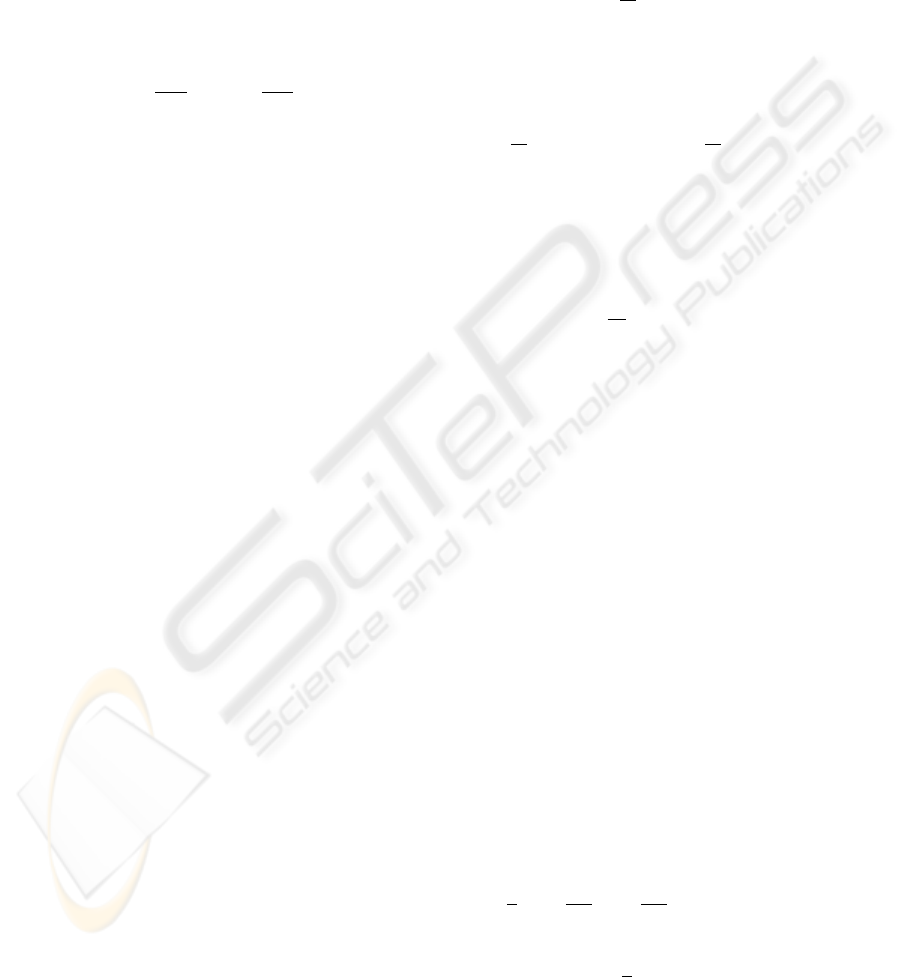From (2) and (3), the sum w
m,k
can be expressed as
w
m,k
=
i
g
o
i
C
col
m+i,k
+
j
g
o
j
C
row
m,k+j
L
l=L
λ
d
l
C
m+l,k
+
L
l=L
λ
e
l
C
m,k+l
(5)
with C
m,k
=
i,j
h
o
i
h
o
j
u
m+i,k+j
. Therefore, a lift-
ing wavelet ﬁlter deﬁned by w
m,k
approximates a par-
tial differential operator L(λ
d
e
) deﬁned by
L(λ
d
e
)u =
2
∂x
2
(I
y
u)+
2
∂y
2
(I
x
u)
I(λ
d
e
)u. (6)
Here I
y
u, I
x
u and I(λ
d
e
)u represent the integral
versions of C
col
m,k
, C
row
m,k
and the last term of (5), re-
spectively, and
λ
d
=(λ
d
L
, ..., λ
d
L
)
e
=(λ
e
L
, ..., λ
e
L
).
3 KURTOSIS-MINIMIZATION
LEARNING
deﬁned in the zero-mean case by the equation
kurt(w)=<w
4
> 3 <w
2
>
2
, (7)
where w is a random variable and <w>denotes
the expectation of w. In case of kurt(w) > 0, the
distribution of w is said to be supergaussian. If w
has a gaussian distribution, then the kurtosis is zero,
i.e., kurt(w)=0. A typical supergaussian probability
density has a shaper peak and longer tails than the
gaussian probability density function (pdf). There-
fore, the variance of gaussian distribution is bigger
than that of supergaussian pdf. It is known that the
value of kurtosis is bounded below. Such properties
of kurtosis are useful for our analysis.
Let us denote a training image also by u
i,j
, and its
domain by
d
. We extract a facial part such as eyes,
nose, and lips from the training image. The region
of the extracted facial part is denoted by ω
d
, and the
number of pixels in ω
d
by P . The facial part is called
positive data, and the image in the region
d
\ ω
d
negative data. The number of pixels in
d
\ ω
d
is
denoted by N. Using these positive and negative data,
we learn free parameters λ
d
l
s and λ
e
l
s appeared in
(5). Our learning method is to minimize the kurtosis
of the lifting wavelet coefﬁcients for the positive data,
and to minimize the variance of those for the negative
data.
We extend the facial part periodically in horizon-
tal and vertical directions, and compute the wavelet
coefﬁcients w
m,k
, deﬁned by (4), of the facial part.
Assume that λ
d
l
s and λ
e
l
s satisfy
L
l=L
(λ
d
l
+ λ
e
l
)=0. (8)
Then, we can prove
<w>=
1
P
(m,k)ω
d
w
m,k
=0. (9)
Therefore, the kurtosis of the lifting wavelet coefﬁ-
cients w
m,k
is given by
J
1
=
1
P
(m,k)ω
d
w
4
m,k
3
1
P
(m,k)ω
d
w
2
m,k
2
,
(10)
which is minimized.
For the negative data in the region
d
\ω
d
, we com-
pute their lifting wavelet coefﬁcients w
m,k
, and mini-
mize the variance
J
2
=
1
N
(m,k)
d
\ω
d
w
2
m,k
. (11)
Thus, our learning algorithm of free parameters λ
d
l
’s
and λ
e
l
s is a process of minimizing the sum of (10)
and (11) under the condition (8).
On the other hand, the continuous version of this
minimization problem is to minimize
ω
L(λ
d
e
)u(x, y)
4
dxdy
3
ω
L(λ
d
e
)u(x, y)
2
dxdy
2
and
\ω
L(λ
d
e
)u(x, y)
2
dxdy
subject to the constraint (8). Here is a region corre-
sponding to
d
, and ω a region corresponding to ω
d
.
The operator L(λ
d
e
) has been given in (6). This
continuous problem is an inverse problem which is
ill-conditioned. Therefore, the discrete version is also
ill-conditioned. To overcome this difﬁculty, we em-
ploy the regularization method. Thus, a functional to
be minimized is provided by
J =
1
4
J
1
+
K
0
4
J
2
+
K
1
2
L
l=L
(λ
d
l
+ λ
e
l
)
2
+
δ
2
L
l=L
(λ
d
l
)
2
+(λ
e
l
)
2
. (12)
Here the third term of the right hand side comes from
(8), and K
i
,i =0, 1 are penalty constants. The last
FACIAL PARTS RECOGNITION USING LIFTING WAVELET FILTERS LEARNED BY
KURTOSIS-MINIMIZATION
43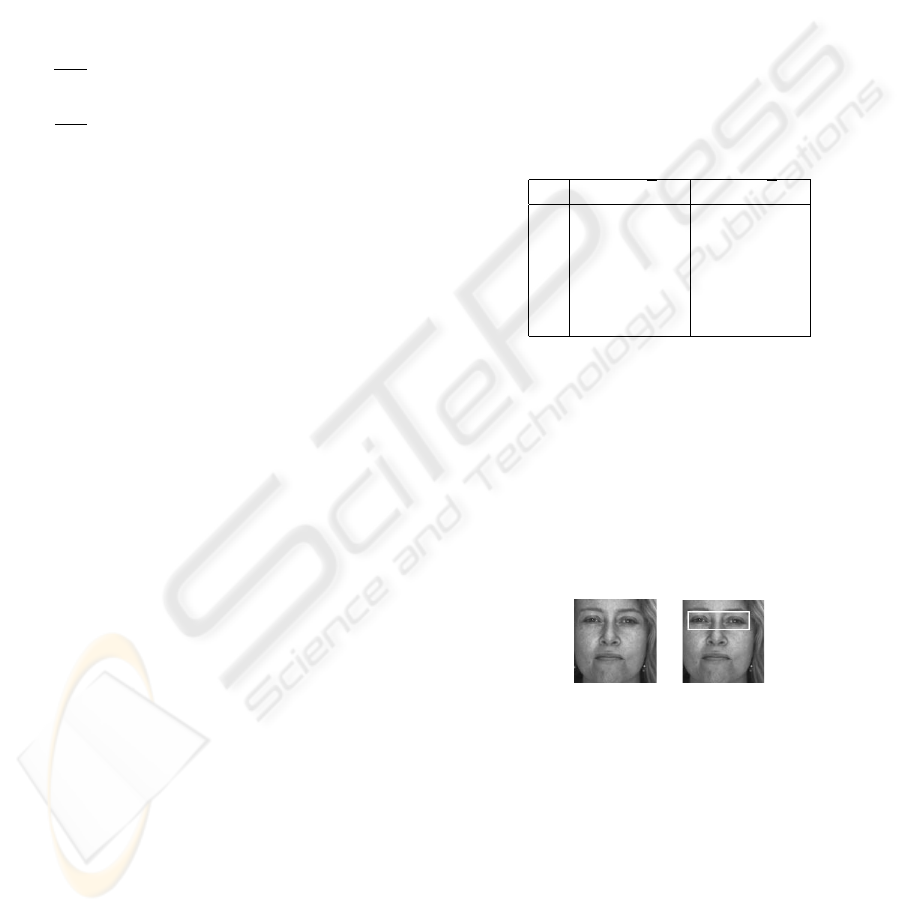term means regularization and δ is a sufﬁciently small
positive number.
Although the functional (12) is a polynomial of
fourth degree with respect to the free parameters λ
d
l
’s
and λ
e
l
s, it has a possibility of having many local
minima. Since it is difﬁcult to obtain a global min-
imum, we seek local minima. Various gradient meth-
ods are often used for computing local minima. How-
ever, these methods are slow in convergence. In this
paper, we employ Newton’s method to solve the prob-
lem fast. Newton’s method is applied to a system of
simultaneous nonlinear equations:
∂J
∂λ
d
l
=0,l= L, ..., L, (13)
∂J
∂λ
e
l
=0,l= L, ..., L. (14)
The process of solving (13) and (14) by Newton’s
method gives our algorithm for learning the free para-
meters λ
d
l
s and λ
e
l
’s.
4 FACIAL PARTS EXTRACTION
The learned parameters λ
d
l
s and λ
e
l
s make the vari-
ance of the lifting wavelet coefﬁcients for the posi-
tive data large, and that for the negative data small.
Therefore, we can extract facial parts of a query im-
age similar to the training facial part by selecting the
large wavelet coefﬁcients, which are computed using
the learned lifting ﬁlter. Our facial extraction algo-
rithm involves the following steps.
1. Compute wavelet coefﬁcients in horizontal and
vertical directions by applying the initial dyadic
wavelet ﬁlters to a query image u
i,j
.
2. By combining them with the parameters λ
d
l
s and
λ
e
l
s learned for the training image, compute the
lifting wavelet coefﬁcients D
m,k
and E
m,k
deﬁned
by (2) and (3), respectively.
3. Calculate the sum w
m,k
= D
m,k
+ E
m,k
.
4. Find the locations (m, k) such that |w
m,k
|≥,
where σ is the standard deviation of lifting wavelet
coefﬁcients computed for the training facial part
and R denotes some constant.
5. Extract an image region, in which the detected lo-
cations are concentrated, as an object similar to the
training facial part.
5 RECOGNIZER
The learned ﬁlter can extract only a facial part sim-
ilar to the training one. For example, if the train-
ing pattern is narrow eyes, it does not extract closed
eyes. Therefore, the learned ﬁlter is a recognizer of
the training facial part. It is important to indicate that
a lifting ﬁlter has to be learned per facial part. The
positive data may be constructed by combining the
same type of several facial parts such as large eyes,
narrow eyes, and closed eyes.
6 SIMULATION
We conducted our experiments on the AR face data-
base (Martinez and Benavente, 1998). The initial ﬁl-
ters we use are the cubic spline dyadic wavelet ﬁl-
ters listed in Table 1 (Mallat, 1998). The number of
Table 1: Cubic spline dyadic wavelet ﬁlters (only low-pass
and high-pass analysis ﬁlters).
n h
o
n
/
2 g
o
n
/
2
-2 0.03125
-1 0.15625
0 0.31250 -0.25
1 0.31250 0.50
2 0.15625 -0.25
3 0.03125
free parameters in each direction is 15, i.e., L =7.
Therefore, 30 free parameters are determined exploit-
ing our learning algorithm. The gray scales of images
are converted into the range [0, 1] for stable computa-
tion.
The training pattern is the facial image of a female
having 120 ×120 size, which is shown in Figure 1(a).
The positive data are her narrow eyes and the nega-
tive data are the image except for the eye region. The
positive data are illustrated in Figure 1(b).
(a) (b)
Figure 1: (a) Training facial image, (b) Positive data of nar-
row eyes.
The penalty constants K
i
,i=0, 1 appeared in (12)
are chosen as K
0
=50and K
1
= 1000, respec-
tively. The regularization constant δ is selected as
δ =0.0001. Newton’s iteration for solving (13) and
(14) was started from zero-vector. We list the learned
parameters in Table 2.
Figure 2 shows the histograms of the wavelet co-
efﬁcients w
m,k
, (m, k) ω
d
obtained exploiting the
initial and the learned lifting ﬁlters. As seen from Fig-
ure 2, the latter histogram is ﬂatter compared with the
VISAPP 2006 - IMAGE UNDERSTANDING
44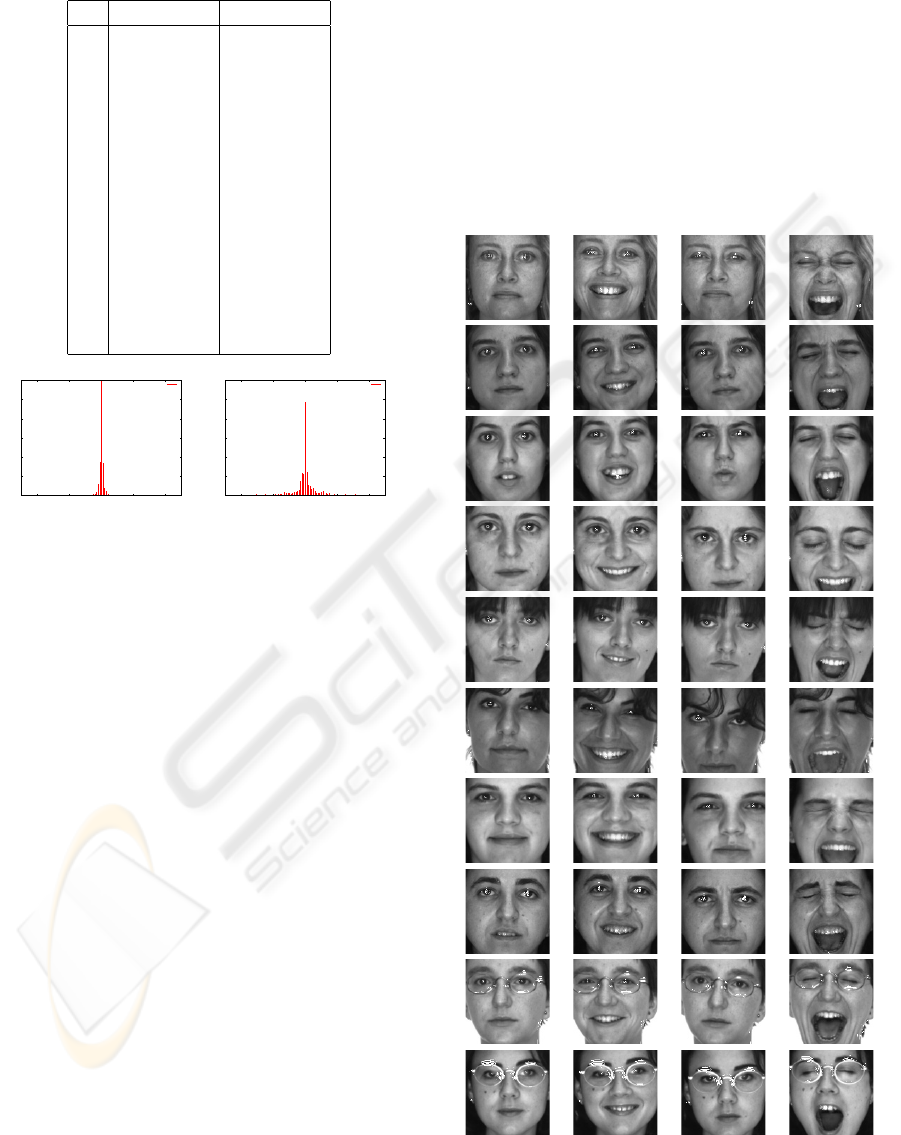Table 2: Learned parameters for the positive and negative
data of the facial image illustrated in Figure 1.
l λ
d
l
λ
e
l
-7 -0.026973 -0.013236
-6 0.103753 0.107106
-5 -0.166372 -0.291907
-4 0.056374 0.200126
-3 0.222947 0.288220
-2 -0.427482 -0.631153
-1 0.760302 0.906046
0 -2.181300 -2.181300
1 3.417086 3.379362
2 -1.808546 -1.953494
3 -0.912882 -0.740206
4 1.503153 1.561924
5 -0.382691 -0.580599
6 -0.360328 -0.224570
7 0.204865 0.171818
0
100
200
300
400
500
600
-40 -20 0 20 40
"hist_org.dat" using 1:2
0
100
200
300
400
500
600
-40 -20 0 20 40
"hist_lift.dat" using 1:2
(a) (b)
Figure 2: Histograms of the wavelet coefﬁcients
w
m,k
, (m, k) ω
d
obtained by using (a) the initial ﬁlter,
(b) the learned lifting ﬁlter.
former one. This means that the variance of the latter
histogram is bigger than that of the former one. Ac-
tually, the standard derivations of the former and the
latter distributions for the positive data were 0.0155
and 0.0770, respectively.
Using the learned ﬁlter, we tried to extract the eyes
from the faces of 10 females and 10 males, whose ex-
pressions involve standard, smile, anger and scream.
The constant R in the extraction algorithm was cho-
sen as R =0.4. Figures 3 and 4 show the experimen-
tal results for the females, and for the males, respec-
tively. We see from Figures 3 and 4 that many of the
narrow eyes have been extracted. In several examples,
a part of the large eyes has been detected, because
it is similar to that of the training narrow eyes. The
learned ﬁlter never extracts the closed eyes. However,
the eyes of persons wearing the glasses can not be ex-
tracted enough using the present learned ﬁlter. The
learned ﬁlter is a recognizer of narrow eyes.
We also tested our algorithm for some illuminated
faces, which contain large eyes. The experimental re-
sults are shown in Figure 5 for the females, and in
Figure 6 for males. Since a part of the training narrow
eyes is similar to that of large eyes, almost all large
eyes have been extracted, independent of illumination
change. The learning time was 1 msec and the detec-
tion time was 0.1 msec per face, by using the laptop
computer with Pentium M, 1.1GHz.
For comparison, we carried out numerical experi-
ments using the variance-maximization method pro-
posed in (Niijima, 2005) for the same faces shown in
Figures 3 through 6. Although the same training and
target images were used, eyebrows, lips and teeth as
well as the narrow eyes were extracted for many of the
faces. For some facial images, the target eyes could
not be extracted.
Figure 3: Narrow eyes extracting results for females.
FACIAL PARTS RECOGNITION USING LIFTING WAVELET FILTERS LEARNED BY
KURTOSIS-MINIMIZATION
45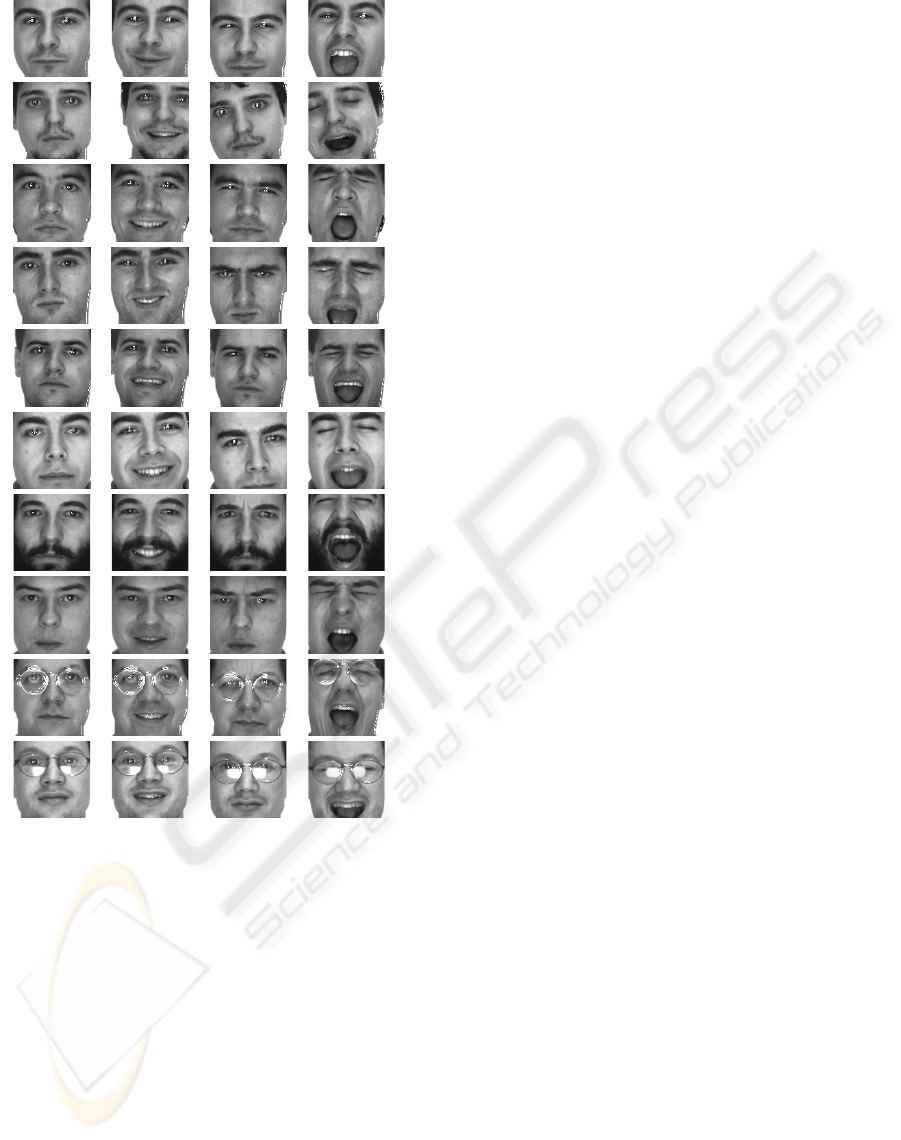Figure 4: Narrow eyes extracting results for males.
7 CONCLUSION
We have proposed a facial parts recognition method.
The method is based on the kurtosis-minimization
learning of the lifting wavelet ﬁlters. Our learning and
recognition algorithms are very fast, because only one
set of free parameters is learned and only one pair of
lifting wavelet ﬁlters with the learned parameters is
applied to a query image for ﬁnding facial parts simi-
lar to the target facial part.
In simulation, we succeeded to extract and recog-
nize the narrow eyes for almost all facial images. The
learned ﬁlter never extracts the closed eyes. Our ﬁl-
ter extracts large eyes for illuminated facial images.
It is a future work to construct a lifting wavelet ﬁlter
recognizable the eyes of a person wearing glasses.
REFERENCES
Abdukirim, T., Niijima, K., and Takano, S. (2005). De-
sign of biorthogonal wavelet ﬁlters using dyadic lift-
ing scheme. In Bulletin of Information and Cybernet-
ics. Kyushu University.
Jaimes, A., Pelz, J., Grabowski, T., Babcock, J., and Chang,
S. (2001). Using human observers’ eye movements
in automatic image classiﬁers. In SPIE Human Vision
and Electronic Imaging.
Mallat, S. (1998). A Wavelet Tour of Signal Processing.
Martinez, A. and Benavente, R. (1998). The ar face data-
base. In CVC Technical Report 24. Purdue University.
Niijima, K. (2005). Fast objects detection by variance-
maximization learning of lifting wavelet ﬁlters.
In SPARS’05, International Workshop on Signal
Processing with Adaptive Sparse Structured Repre-
sentation.
Pentland, A., Moghaddam, B., and Starner, T. (1994).
View-based and modular eigenspaces for face recog-
nition. In CVPR’94, IEEE Conference on Computer
Vision and Pattern Recognition.
Sweldens, W. (1996). The lifting scheme: A custom-design
construction of bi-orthogonal wavelets. In Applied
and Computational Harmonic Analysis. Elsevier.
Takano, S. and Niijima, K. (2005). Person identiﬁcation
using fast face learning of lifting dyadic wavelet ﬁl-
ters. In CORES’05, 4th International Conference on
Computer Recognition Systems. Springer.
Takano, S., Niijima, K., and Abudukirim, T. (2003). Fast
face detection by lifting dyadic wavelet ﬁlters. In
ICIP’03, IEEE International Conference on Image
Processing.
Takano, S., Niijima, K., and Kuzume, K. (2004). Per-
sonal identiﬁcation by multiresolution analysis of lift-
ing dyadic wavelets. In EUSIPCO’04, 12th European
Signal Processing Conference.
Tieu, K. and Viola, P. (2000). Boosting image retrieval.
In CVPR2000, IEEE Conference on Computer Vision
and Pattern Recognition.
Vapnik, V. (1998). Statistical Learning Theory. John Wiley
& Sons Inc., New York.
VISAPP 2006 - IMAGE UNDERSTANDING
46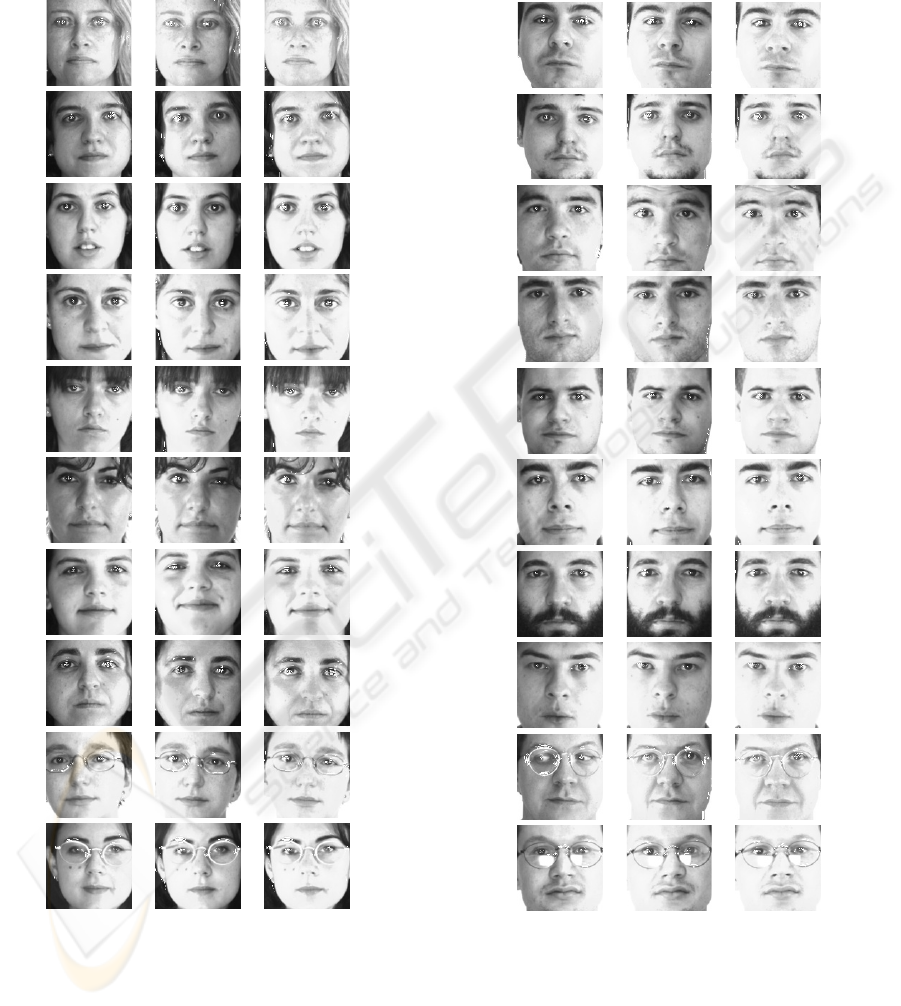Figure 5: Extraction results for the illuminated faces of fe-
males.
Figure 6: Extraction results for the illuminated faces of
males.
FACIAL PARTS RECOGNITION USING LIFTING WAVELET FILTERS LEARNED BY
KURTOSIS-MINIMIZATION
47